## Spatial Simulations for Population Genetics in R & SLiM

### Overview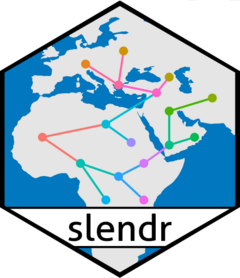slendr is an R package for simulating spatially-explicit genomic data. It allows to programmatically and visually encode spatial population boundaries and temporal dynamics across a geographic landscape (leveraging real cartographic data or abstract, user-defined landscapes), and specify population divergences and geneflow events based on an arbitrary graph of demographic history.

Its strength comes from combining the flexibility of R with its wealth of libraries for graphics, geospatial analysis and statistics, with the power of the forward population genetics simulator SLiM and its massive library of features for simulating genomic data.

The goal of slendr is to provide a convenient R interface for defining arbitrary complex spatial (and non-spatial) models of population history and execute them automatically by SLiM as a backend engine, all without having to leave the convenience of the R environment. By default, output data is saved in a tree-sequence format, allowing efficient simulations of genome-scale and population-scale data. The R package also provides basic functionality for processing the simulated tree-sequence outputs and for calculating the most frequently used population genetics statistics by implementing an interface to the tree-sequence library tskit via the reticulate package. slendr can also serve as a tool for simulating data from non-spatial models, making it possible to write entire simulation and analytic pipelines entirely from R.

This software is still under development! We have been making steady progress towards the first beta version, but the package still has some way to go before being production ready. If you are interested, you can see the list of changes between versions here.

That said, if you would like to learn more, or if you’re feeling brave and would like to test the package yourself, take a look at some of our tutorial vignettes (either the main tutorial or other vignettes available under the “Articles” menu on the project website).

1. Click on the “Watch” button on the project’s Github website.

### Installation

For installation instructions, please take a look at the installation section of the main tutorial. Note that in order to simulate data, you will need the most recent version of the SLiM software (version 3.6 or later). Similarly, if you would like to process tree-sequence data generated by slendr & SLiM from R, you will need a working Python environment with the modules tskit and pyslim (see the relevant vignette for more detail).

The main dependency of slendr is the R package sf, which it uses for manipulation of geospatial data. In turn, sf depends on several external pieces of software which sometimes need to be installed. That said, if you can successfully install sf by running install.packages("sf") you will be good to go. In case you run into trouble, following sf’s installation guidelines has so far always been a success.

### Example

Here is a small demonstration of what slendr is designed to do. We want to simulate spatiotemporal data representing the history of modern humans in Eurasia after the Out of Africa migration. This example will be quite brief, for more details, please see the tutorial vignette.

#### 1. Setup the spatial context

First, we define the spatial context of the simulation. This will be the entire “world” which will be occupied by populations in our model. Note that in the world definition, we are explicitly stating which projected Coordinate Reference System (CRS) will be used to represent landscape features, distances in kilometers, etc.

library(slendr)

map <- world(
xrange = c(-15, 60), # min-max longitude
yrange = c(20, 65),  # min-max latitude
crs = "EPSG:3035"    # real projected CRS used internally
)

We can visualize the defined world map using the generic function plot provided by the package.

plot(map)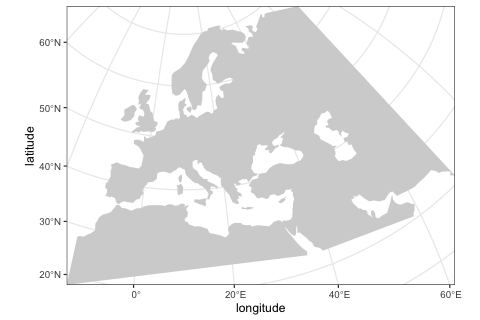Although in this example we use a real Earth landscape, the map can be completely abstract (either blank or with user-defined landscape features such as continents, islands, corridors and barriers).

#### 2. Define broader geographic regions

In order to make building of population boundaries easier, we can define smaller regions on the map using the function region.

Note all coordinates of are specified in the geographic coordinate system (degrees longitude and latitude), but are internally represented in a projected CRS. This makes it easier to define spatial features simply by reading the coordinates from any regular map but makes simulations more accurate (distances and shapes are not distorted because we can use a CRS tailored to the region of the world we are working with).

africa <- region(
"Africa", map,
polygon = list(c(-18, 20), c(40, 20), c(30, 33),
c(20, 32), c(10, 35), c(-8, 35))
)
europe <- region(
"Europe", map,
polygon = list(
c(-8, 35), c(-5, 36), c(10, 38), c(20, 35), c(25, 35),
c(33, 45), c(20, 58), c(-5, 60), c(-15, 50)
)
)
anatolia <- region(
"Anatolia", map,
polygon = list(c(28, 35), c(40, 35), c(42, 40),
c(30, 43), c(27, 40), c(25, 38))
)

Again, we can use the generic plot function to visualize the objects:

plot(africa, europe, anatolia)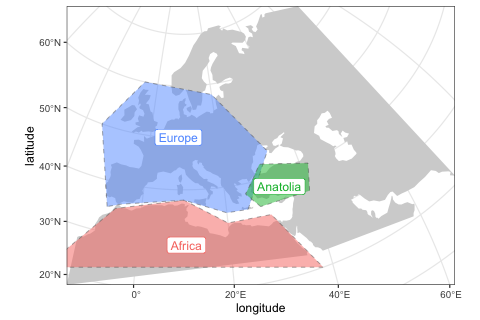#### 3. Define demographic history and population boundaries

The most important function in the package is population, which is used to define names, split times, sizes and spatial ranges of populations. Here, we specify times in years before the present, distances in kilometers. If this makes more sense for your models, times can also be given in a forward direction.

You will also note functions such as move or expand which are designed to take a slendr population object and change its spatial dynamics.

Note that in order to make this example executable on a normal local machine, we deliberately decreased the sizes of all populations.

afr <- population( # African ancestral population
"AFR", parent = "ancestor", time = 52000, N = 3000,
map = map, polygon = africa
)

ooa <- population( # population of the first migrants out of Africa
"OOA", parent = afr, time = 51000, N = 500, remove = 25000,
center = c(33, 30), radius = 400e3
) %>%
move(
trajectory = list(c(40, 30), c(50, 30), c(60, 40)),
start = 50000, end = 40000, snapshots = 20
)

ehg <- population( # Eastern hunter-gatherers
"EHG", parent = ooa, time = 28000, N = 1000, remove = 6000,
polygon = list(
c(26, 55), c(38, 53), c(48, 53), c(60, 53),
c(60, 60), c(48, 63), c(38, 63), c(26, 60))
)

eur <- population( # European population
name = "EUR", parent = ehg, time = 25000, N = 2000,
polygon = europe
)

ana <- population( # Anatolian farmers
name = "ANA", time = 28000, N = 3000, parent = ooa, remove = 4000,
center = c(34, 38), radius = 500e3, polygon = anatolia
) %>%
expand( # expand the range by 2.500 km
by = 2500e3, start = 10000, end = 7000,
polygon = join(europe, anatolia), snapshots = 20
)

yam <- population( # Yamnaya steppe population
name = "YAM", time = 7000, N = 500, parent = ehg, remove = 2500,
polygon = list(c(26, 50), c(38, 49), c(48, 50),
c(48, 56), c(38, 59), c(26, 56))
) %>%
move(trajectory = list(c(15, 50)), start = 5000, end = 3000, snapshots = 10)

We can use the function plot again, but we get a warning informing us that plotting complex model dynamics over time on a single map is not a good idea. Below, we show a better way to do this using a built-in interactive R shiny app.

plot(afr, ooa, ehg, eur, ana, yam)
#> Warning: Attempting to plot population ranges at multiple time points on
#> a single map. This is very hard to do in a satisfying way. Please
#> consider using the function explore() to plot the model dynamics
#> interactively.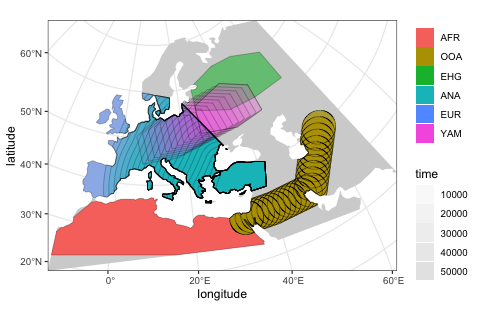#### 4. Define geneflow events

By default, overlapping populations in SLiM do not mix. In order to schedule an geneflow event between two populations, we can use the function geneflow. If we want to specify multiple such events at once, we can collect them in a simple R list:

gf <- list(
geneflow(from = ana, to = yam, rate = 0.5, start = 6500, end = 6400, overlap = FALSE),
geneflow(from = ana, to = eur, rate = 0.5, start = 8000, end = 6000),
geneflow(from = yam, to = eur, rate = 0.75, start = 4000, end = 3000)
)

#### 5. Compile the model to a set of configuration files

model <- compile(
populations = list(afr, ooa, ehg, eur, ana, yam), # populations defined above
geneflow = gf, # geneflow events defined above
generation_time = 30,
resolution = 10e3, # resolution in meters per pixel
competition_dist = 130e3, mate_dist = 100e3, # spatial interaction in SLiM
dispersal_dist = 70e3, # how far will offspring end up from their parents
dir = file.path(tempdir(), "readme-model"), overwrite = TRUE
)

Compiled model is kept as an R object which can be passed to different functions, most importantly the slim() function shown below.

#### 6. Visualize the model

The package provides an R shiny-based browser app explore() for checking the model dynamics interactively and visually. For more complex models, this is much better than static spatial plots such as the one we showed in step 2 above:

explore(model)

The function has two modes:

a) Plotting spatial map dynamics:b) Displaying the demographic history graph (splits and geneflow events) embedded in the specified model:#### 7. Run the model in SLiM

Finally, we can execute the compiled model in SLiM. Here we run the simulation in a batch mode, but we could also run it in SLiMgui by setting method = "gui".

The slim function generates a complete SLiM script tailored to run the spatial model we defined above. This saves you, the user, a tremendous amount of time.

slim(
model,
seq_length = 1, recomb_rate = 0, # simulate only a single locus
save_locations = TRUE, # save the location of everyone who ever lived
method = "batch", # change to "gui" to execute the model in SLiMgui
seed = 314159
)

As specified, the SLiM run will save ancestry proportions in each population over time as well as the location of every individual who ever lived.

#### 8. Re-capitulate the SLiM run as an individual-based animation

We can use the saved locations of every individual that lived throughout the course of the simulation to generate a simple GIF animation:

animate(model, steps = 100, width = 500, height = 300)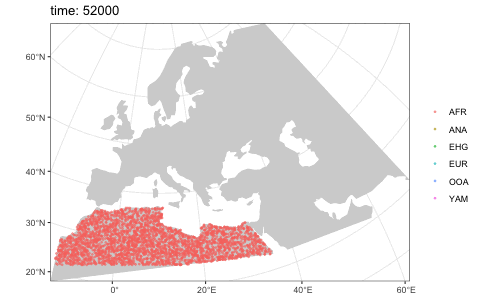Note that it is possible to simulate population splits and geneflows both by “physically” moving individuals of a population from one destination to the next across space but it is also possible to do this more abstractly (in instantaneous “jumps”) in situations where this is more appropriate or where simulating accurate movement is not necessary.

In this case, the only population movement we explicitly encoded was the split and migration of the OOA population from Africans and the expansion of Anatolians. All the other populations simply popped up on a map, without explicitly migrating there. Similarly, the geneflow from Anatolians into the Yamnaya specified in step 4. did not require a spatial overlap between the two populations, but the other two geneflow events did.

## Further information

The example above provides only a very brief overview of the functionality of the slendr package. There is much more to slendr than what we demonstrated here. For instance:

• You can tweak parameters influencing dispersal dynamics (how “clumpy” populations are, how far can offspring migrate from their parents, etc.) and define how these should change over time. For instance, you can see that in the animation above, the African population forms a single “blob” that really isn’t spread out across its entire population range. Tweaking the dispersal parameters as show this vignette helps avoid that.

• You can use slendr to program non-spatial models, which means that any concievable demographic model can be simulated with only a few lines of R code and, for instance, plugged into an Approximate Bayesian Computation pipeline or other use any other R package for downstream analysis in the same R script. You can find more in this vignette.

• You can build complex spatial models which are still abstract (not assuming any real geographic location), including traditional simulations of demes in a lattice structure. A complete example is shown this vignette.

• Because slendr & SLiM save data in a tree-sequence file format, thanks to the R package reticulate for interfacing with Python code, we have the incredible power of tskit and pyslim for manipulating tree-sequence data right at our fingertips, all within the convenient environment of R.